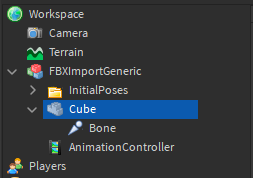# Skinned meshes not deforming right

I want to create a 2D plane where if I move a bone up the bones around it deform to reach the bone I moved up however when I import a basic plane from blender

and the run this script

``````local Cube = workspace.FBXImportGeneric.Cube

for Number = -Cube.Size.X / 2, Cube.Size.X / 2, 2 do
for Number2 = -Cube.Size.Z / 2, Cube.Size.Z / 2, 2 do
local Bone = Instance.new("Bone")
Bone.Visible = true
Bone.CFrame = CFrame.new(Number, Cube.Size.Y / 2, Number2)
Bone.Parent = Cube
end
wait()
end
``````

I get this result

All those blue things are the bones and you can see the cyan boundary line and where the mesh tries to for a illusion of a 3D cube. I don’t know why this is happening?

### If anyone can help that would be much appreciated!

my workspace